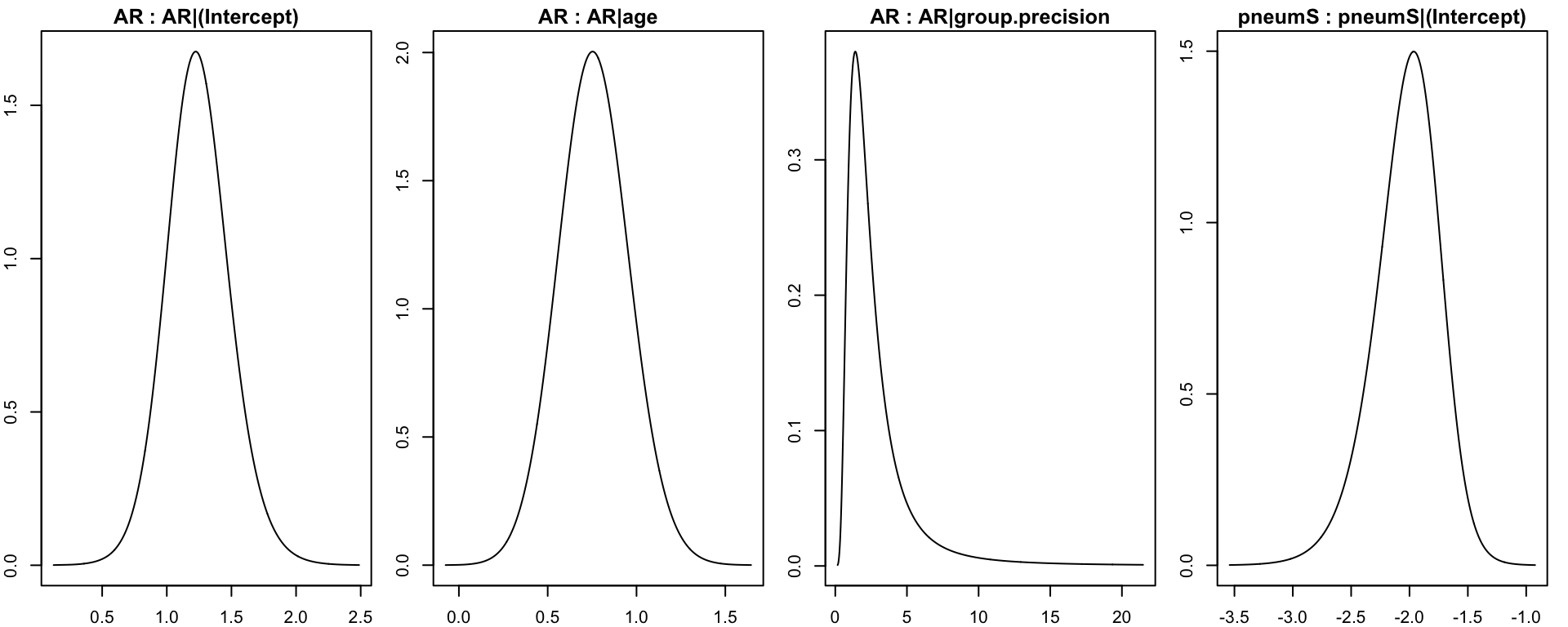Below is the hands-on exercises on the advanced methods. This will cover the heuristic search, how to handle clustering and how to perform Bayesian model averaging.

Note: in the following text: Bayesian network, structure and DAG are synonyms.

# Mixed models – correction for grouped data (or clustering)

Note: In the following: clustering and grouped are taken as synonym.

In some situations, the way the data were collected has a clear grouping aspect, and therefore there is a potential risk for non-independence between data points from the same group that could cause over-dispersion. This can lead to analyses which are over-optimistic as the true level of variation in the data is under-estimated. It could have an impact … or not. But this is a good practice to check!

In practice we will introduce a random effect to account for this additional variability. Thus each node will become a GLMM (Generalized Linear Mixed Model (Faraway, 2016)) instead of a GLM (Generalized Linear Model (McCullagh, 2018)) but in a Bayesian setting. We will then compute the posterior distribution and check if they widen. In such case, we will have to take into account the clustering in the scoring scheme we use.

In practice, the major problem is the computational complexity of this approach. Indeed, if the clustering do not affect too much the result, it is preferable to not take it into account. The model is then much simpler and parsimonious then more generalizable.

The grouping variable is `farm` and we apply the random effect to every nodes. On a personal computer following code takes 15 minutes where the previous code ran in less than a second! The grouping variable has 15 levels.

``````marg.f.grouped <- fitabn(dag.m = trim.dag,
data.df = as.data.frame(abndata),
data.dists = dist,
group.var = "farm",
cor.vars = c("AR", "pneumS", "female", "livdam", "eggs", "wormCount", "age", "adg"),
compute.fixed = TRUE,
n.grid = 1000)``````

## Visually inspect the marginal posterior distributions of the parameters